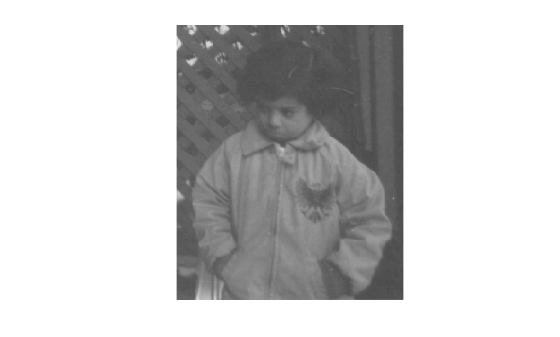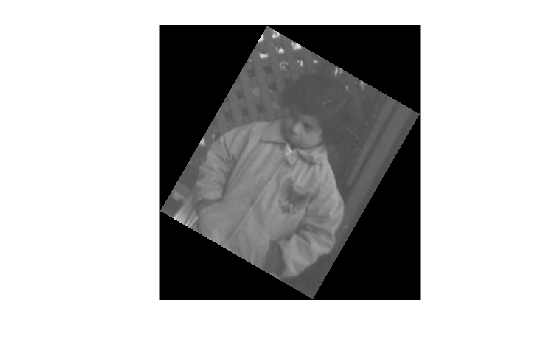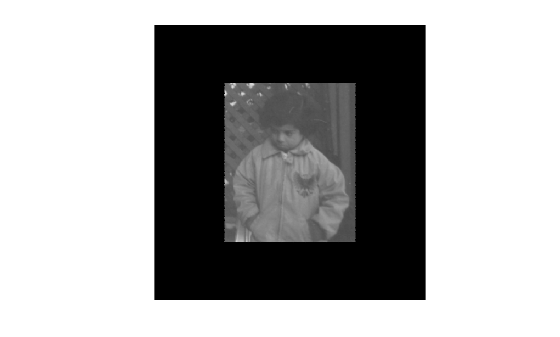# invert

Invert geometric transformation

## Syntax

``tformInv = invert(tform)``

## Description

example

````tformInv = invert(tform)` returns the inverse of the geometric transformation `tform`.```

## Examples

collapse all

```I = imread("pout.tif"); imshow(I)```Create a `rigidtform2d` object that defines a 30 degree clockwise rotation around the origin.

```translation = [0 0]; theta = 30; tform = rigidtform2d(theta,translation);```

Apply the forward geometric transformation, `tform`, to the image. Display the rotated image.

```J = imwarp(I,tform); imshow(J)```Invert the geometric transformation. The result is a new `rigidtform2d` object that represents a 30 degree rotation in the counterclockwise direction.

`invtform = invert(tform);`

Apply the inverse geometric transformation, `invtform`, to the rotated image `J`. The final image, `K`, has the same size, shape, and orientation as the original image. Black padding around the image results from the two transformations.

```K = imwarp(J,invtform); imshow(K)```## Input Arguments

collapse all

Geometric transformation, specified as a geometric transformation object listed in the table.

Geometric Transformation ObjectDescription
2-D Geometric Transformations
`transltform2d`Translation transformation
`rigidtform2d`Rigid transformation: translation and rotation
`simtform2d`Similarity transformation: translation, rotation, and isotropic scaling
`affinetform2d`Affine transformation: translation, rotation, anisotropic scaling, reflection, and shearing
`projtform2d`Projective transformation
3-D Geometric Transformations
`transltform3d`Translation transformation
`rigidtform3d`Rigid transformation: translation and rotation
`simtform3d`Similarity transformation: translation, rotation, and isotropic scaling
`affinetform3d`Affine transformation: translation, rotation, anisotropic scaling, reflection, and shearing

Note

You can also specify `tform` as an object of type `rigid2d`, `rigid3d`, `affine2d`, `affine3d`, or `projective2d`. However, these objects are not recommended. For more information, see Compatibility Considerations.

## Output Arguments

collapse all

Inverse geometric transformation, returned as a geometric transformation object of the same type as `tform`.

## Version History

Introduced in R2013a

expand all